# Lesson 3 6 Implicit Differentiation Objectives Use implicit

• Slides: 11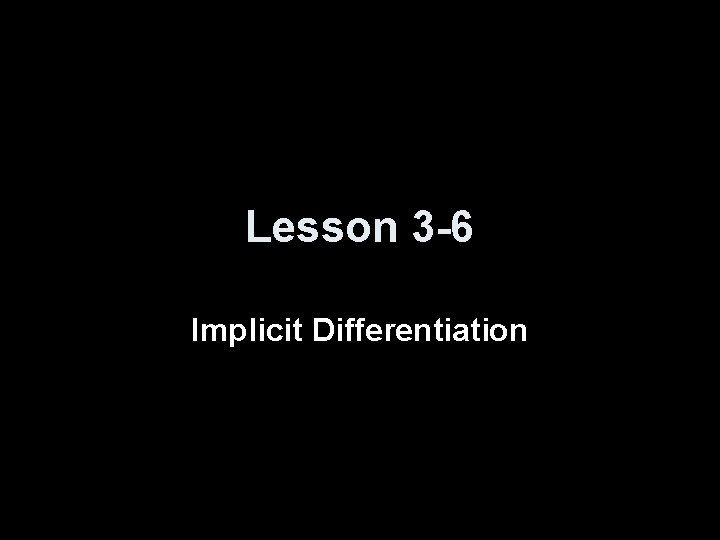Lesson 3 -6 Implicit Differentiation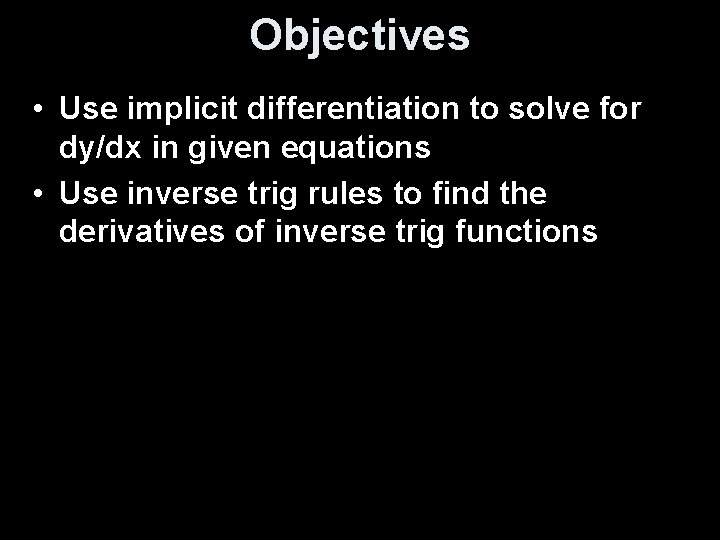Objectives • Use implicit differentiation to solve for dy/dx in given equations • Use inverse trig rules to find the derivatives of inverse trig functionsVocabulary • Implicit Differentiation – differentiating both sides of an equation with respect to one variable and then solving for the other variable “prime” (derivative with respect to the first variable) • Orthogonal – curves are orthogonal if their tangent lines are perpendicular at each point of intersection • Orthogonal trajectories – are families of curves that are orthogonal to every curve in the other family (lots of applications in physics (example: lines of force and lines of constant potential in electricity)Implicit Differentiation If a function (or a relation) can not be set into the for y = f(x), then implicit differentiation (differentiating both sides with respect to x and solving for y’) can be used to find the derivative. Example: (a circle with radius 10, which is a relation and not a function) x² + y² = 100 dx dy dy 2 x --- + 2 y ---- = 0 2 x + 2 y ---- = 0 dx dx dx dy 2 x = -2 y ---dx dy -x ---- = ----dx y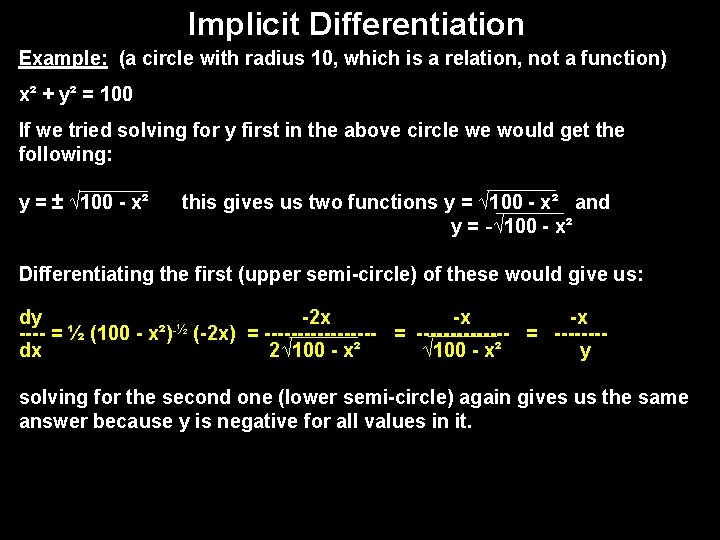Implicit Differentiation Example: (a circle with radius 10, which is a relation, not a function) x² + y² = 100 If we tried solving for y first in the above circle we would get the following: y = ± √ 100 - x² this gives us two functions y = √ 100 - x² and y = -√ 100 - x² Differentiating the first (upper semi-circle) of these would give us: dy -2 x -x -x -½ ---- = ½ (100 - x²) (-2 x) = --------- = -------dx 2√ 100 - x² y solving for the second one (lower semi-circle) again gives us the same answer because y is negative for all values in it.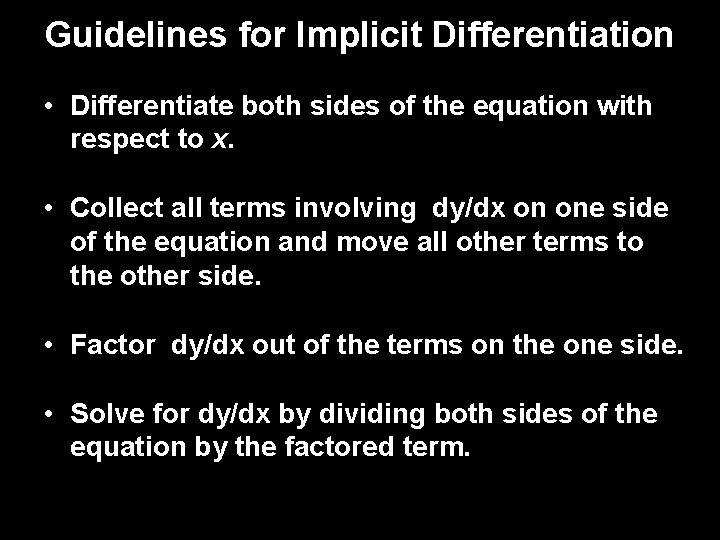Guidelines for Implicit Differentiation • Differentiate both sides of the equation with respect to x. • Collect all terms involving dy/dx on one side of the equation and move all other terms to the other side. • Factor dy/dx out of the terms on the one side. • Solve for dy/dx by dividing both sides of the equation by the factored term.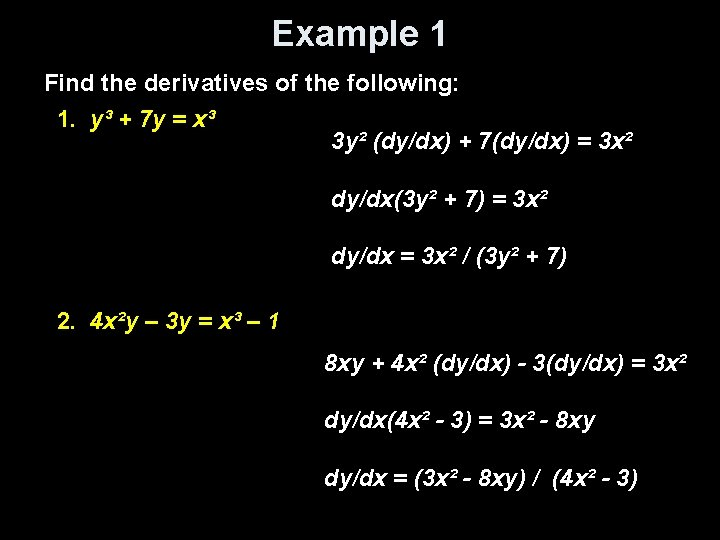Example 1 Find the derivatives of the following: 1. y³ + 7 y = x³ 3 y² (dy/dx) + 7(dy/dx) = 3 x² dy/dx(3 y² + 7) = 3 x² dy/dx = 3 x² / (3 y² + 7) 2. 4 x²y – 3 y = x³ – 1 8 xy + 4 x² (dy/dx) - 3(dy/dx) = 3 x² dy/dx(4 x² - 3) = 3 x² - 8 xy dy/dx = (3 x² - 8 xy) / (4 x² - 3)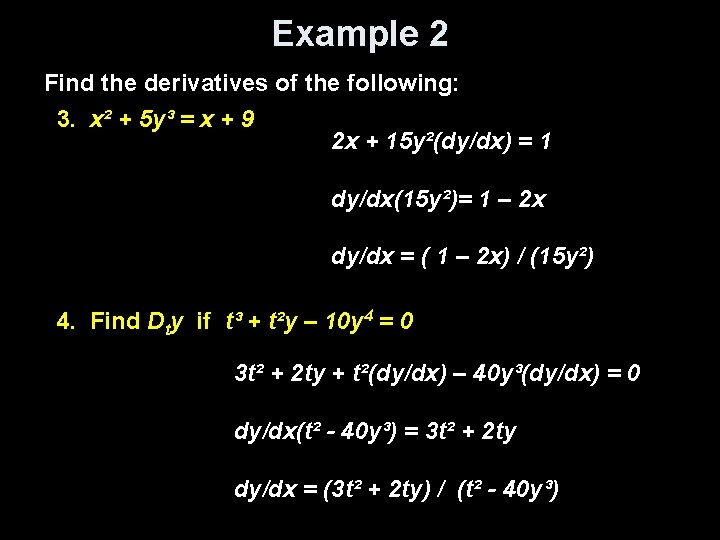Example 2 Find the derivatives of the following: 3. x² + 5 y³ = x + 9 2 x + 15 y²(dy/dx) = 1 dy/dx(15 y²)= 1 – 2 x dy/dx = ( 1 – 2 x) / (15 y²) 4. Find Dty if t³ + t²y – 10 y 4 = 0 3 t² + 2 ty + t²(dy/dx) – 40 y³(dy/dx) = 0 dy/dx(t² - 40 y³) = 3 t² + 2 ty dy/dx = (3 t² + 2 ty) / (t² - 40 y³)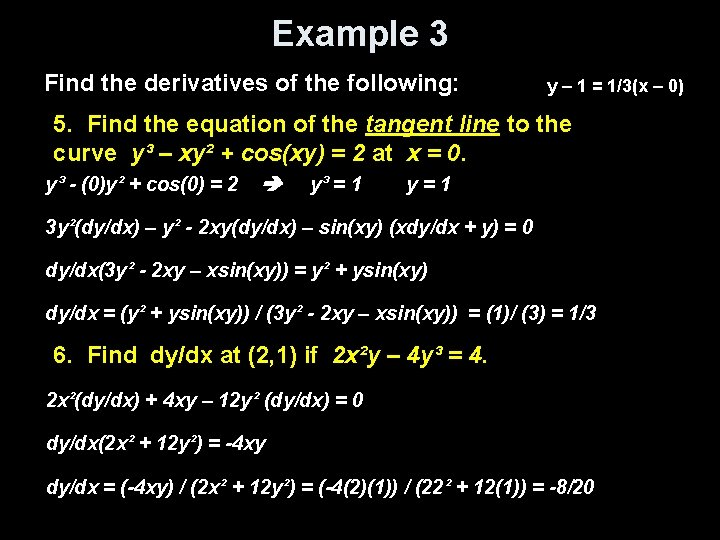Example 3 Find the derivatives of the following: y – 1 = 1/3(x – 0) 5. Find the equation of the tangent line to the curve y³ – xy² + cos(xy) = 2 at x = 0. y³ - (0)y² + cos(0) = 2 y³ = 1 y=1 3 y²(dy/dx) – y² - 2 xy(dy/dx) – sin(xy) (xdy/dx + y) = 0 dy/dx(3 y² - 2 xy – xsin(xy)) = y² + ysin(xy) dy/dx = (y² + ysin(xy)) / (3 y² - 2 xy – xsin(xy)) = (1)/ (3) = 1/3 6. Find dy/dx at (2, 1) if 2 x²y – 4 y³ = 4. 2 x²(dy/dx) + 4 xy – 12 y² (dy/dx) = 0 dy/dx(2 x² + 12 y²) = -4 xy dy/dx = (-4 xy) / (2 x² + 12 y²) = (-4(2)(1)) / (22² + 12(1)) = -8/20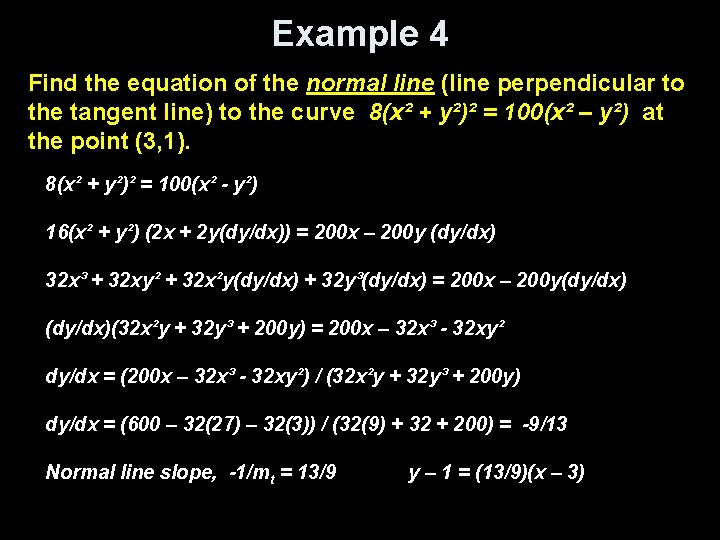Example 4 Find the equation of the normal line (line perpendicular to the tangent line) to the curve 8(x² + y²)² = 100(x² – y²) at the point (3, 1). 8(x² + y²)² = 100(x² - y²) 16(x² + y²) (2 x + 2 y(dy/dx)) = 200 x – 200 y (dy/dx) 32 x³ + 32 xy² + 32 x²y(dy/dx) + 32 y³(dy/dx) = 200 x – 200 y(dy/dx)(32 x²y + 32 y³ + 200 y) = 200 x – 32 x³ - 32 xy² dy/dx = (200 x – 32 x³ - 32 xy²) / (32 x²y + 32 y³ + 200 y) dy/dx = (600 – 32(27) – 32(3)) / (32(9) + 32 + 200) = -9/13 Normal line slope, -1/mt = 13/9 y – 1 = (13/9)(x – 3)Summary & Homework • Summary: – Use implicit differentiation when equation can’t be solved for y = f(x) – Derivatives of inverse trig functions do not involve trig functions • Homework: – pg 233 -235: 1, 6, 7, 11, 17, 25, 41, 47